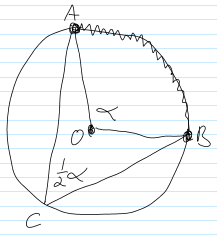$\def\bbN{{\mathbb N}} \def\bbQ{{\mathbb Q}} \def\bbR{{\mathbb R}} \def\bbZ{{\mathbb Z}}$
 © | Dror Bar-Natan: Classes: 2014-15: Math 475 - Problem Solving Seminar: (27) Next: Blackboards for Thursday February 12 Previous: Quiz #5 - Exploit Symmetry

# Handout for February 12, "Exploit Symmetry" and "Divide into Cases"

Reading. Sections 1.6 and 1.7 of Larson's textbook.

Next Quiz. Tuesday February 24, on these two sections.

Problem 1. Prove: You cannot colour the points of the plane with just three colours, so that no two points of distance 1 will be coloured with the same colour. What if you had four colours available?

Problem 2 (Larson's 1.6.2).

1. Of all the rectangles which can be inscribed in a given circle, which has the greatest area?
2. Maximize $\sin\alpha+\sin\beta+\sin\gamma$, where $\alpha,\beta,\gamma$ are the angles of a triangle.
3. Of all the triangles of a fixed perimeter, which has the greatest area?
4. Of all the parallelepipeds of volume 1, which has the smallest surface area?
5. Of all the $n$-gons which can be inscribed in a given circle, which has the greatest area?
6. Dror adds: of all the $n$-gons with a given perimeter, which has the greatest area?Problem 3 (Larson's 1.7.1). Prove that an angle inscribed in a circle is equal to one half the central angle which subtends the same arc, as in the picture on the right.

Problem 4 (Larson's 1.7.8). Determine $F(x)$, if for all real $x$ and $y$, $F(x)F(y)-F(xy)=x+y$.